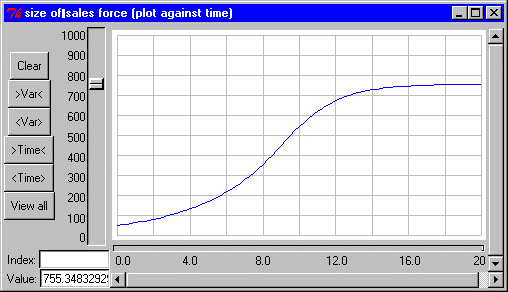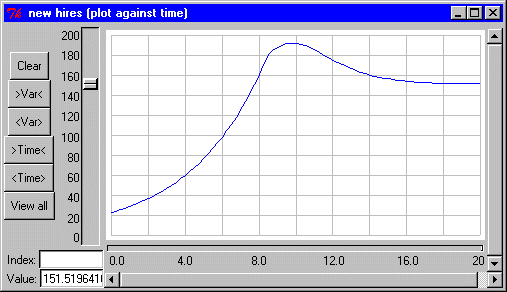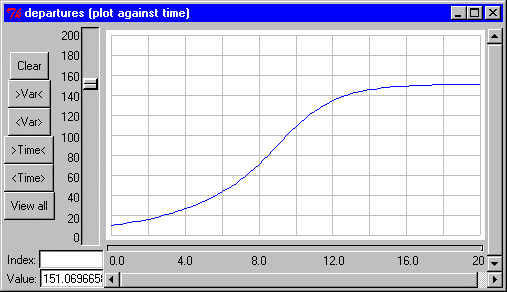# Lumped model ;

## S-shaped (logistic) growth of a company

ModelId:
cogrowth1
SimileVersion:
3.1+

This models the changing size of the sales force of a company, using feedbacks between the size of the sales force, the success of this sales force in selling goods, and further recruitment.

Source: Andrew Ford (1999): Modelling the Environment, p.64Equations:

Compartments:

size of sales force
Initial value = 50
Rate of change = + new_hires - departures

Flows:
new hires = hiring_fraction*(budgeted_size_of_sales_force-size_of_sales_force)
departures = size_of_sales_force*exit_rate

Variables:
annual revenue in millions = widget_sales*widget_price/1000000
budgeted size of sales force = sales_dept_budget*1000000/average_salary
effectiveness in widgets per day = graph(size_of_sales_force)
sales dept budget = fraction_to_sales*annual_revenue_in_millions
widget sales = size_of_sales_force*effectiveness_in_widgets_per_day*365

Parameters:
average salary = 25000
exit rate = 0.2
fraction to sales = 0.5
hiring fraction = 1
widget price = 100

Results:References:

Ford, A (1999) Modeling the Environment.

Model tags: